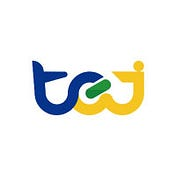# 【Application(13)】Momentum select

along for the ride

# Highlights

• Difficulty：★★☆☆☆

# Preface

we introduce the Momentum trade last time ,now we are going to teach you how to find this stock ,if we are not highly involved the marker,we often found in the news which already price in ,so what we want to do is find the stock automatic.It also can save our time .

we write the function more flexible.you can change the parameter by yourself ! Following is the way to our set the parameter :

1. when the trading volume is 5 times more than the average of previous 4 days.
2. stock price rise at least 3%
3. the trade volume is more than 500

# The Editing Environment and Modules Required

Window10 Spyder(anaconda31)

# Database

Security Transaction Data Table：Listed securities with unadjusted price and index. Code is ‘TWN/EWPRCD’

Securities attribute information Data Table : Listed securities industry category and name， Code is 'TWN/ANPRCSTD'

`import pandas as pdimport matplotlib.pyplot as pltimport numpy as np#################TEJimport tejapitejapi.ApiConfig.api_key = 'Your Key'tejapi.ApiConfig.ignoretz = True###############draw k line from mplfinance.original_flavor import candlestick_ohlcfrom matplotlib.dates import date2num ,num2dateimport matplotlib.ticker as tickerimport matplotlib.dates`

# Data Selection

`data=tejapi.get('TWN/EWNPRCSTD' ,chinese_column_name=True )select=data["上市別"].unique()`
`select=select[1:3]condition =(data["上市別"].isin(select)) & ( data["證券種類名稱"]=="普通股" )data=data[condition] ######設條件twid=data["證券碼"].to_list()`

select [‘coid’,’open_d’,’close_d’,’high_d’,’low_d’ ,’mdate’, ‘volume’, ‘close_adj’ ]

`opts={'columns': ['coid','open_d','close_d','high_d','low_d' ,'mdate', 'volume', 'close_adj']}start='2022-1-01'end="2022-03-8"tw=tejapi.get('TWN/EWPRCD',coid=twid,                mdate={'gt':start,'lt':end},                paginate=True,                        chinese_column_name=True,                opts=opts                )a=twa=tw.groupby(by=["證券碼"])b=list(a) #######ˇ`

use the pandas groupby function , and we have to put there into a list ,so we can observe the data

we should open it

# Data Processing

Step 1. set the indicator

`def selectstock(b,number,minnum,num) : # b為用groupby做出來的list    output=[]    for i in range(len(b)):        a=b[i]        a["五日均量"]=a["成交量(千股)"].rolling(5).sum()        a["五日均價"]=a["收盤價-除權息"].rolling(5).mean()                a["前幾日平均"]=(a["五日均量"]-a["成交量(千股)"]) / 4        a["成交量"+str(number)+"倍喔"]=a["成交量(千股)"]-a['前幾日平均'] *number         a.drop('五日均量',axis=1)        if a["成交量(千股)"].mean() > minnum  :            output.append(a)    stockineed=[]for j in output:        j.reset_index(drop=True,inplace=True)        if j["成交量"+str(number)+"倍喔"][len(j)-1] > 0 :                        if j["收盤價-除權息"][len(j)-1] > j["收盤價-除權息"][len(j)-2]*num :                stock=j["證券碼"]                stockineed.append(stock)    return stockineed`

this function have 3 parameter (number,minnum,num)

number : select 5 times more than previous four day

minnum : the trade volume minium we select ”500"

num : stock price growth percentage we select 3 %

`stockineed=selectstock(b,5,500,1.03)`

there is 8 stocks confrom to our conditions

`condition=tw["證券碼"].isin(stockineed)tw1=tw[condition]`
`a=tw1.groupby("證券碼")a=list(a)  for i in a:    i.set_index("日期",inplace=True)  ###set date to index`

use the groupby function again and we can see each group of the stcok

# Step 2 visualize the stocks

we try to get the stock name and the catagory in other database ,so we can combine all of them in one picture

`opts={'columns': ['coid','stk_name','mkt','tejindnm',]}data1=tejapi.get('TWN/ANPRCSTD' ,                opts=opts,                chinese_column_name=True,paginate=True,                coid=stockineed)`
`def getplot(finaldata):for i in range(len(finaldata)):                out=finaldata[i]        name=data1[data1["證券碼"]==finaldata[i]]                outputname0=name.loc[i,'證券名稱']##為了得到標題        outputname1=name.loc[i,'上市別'] ##        outputname2=name.loc[i,"TEJ產業名"]##        # out.reset_index(drop=True,inplace=True)        if outputname1 == "TSE":            outputname1 ="上市"        else :            outputname1= "上櫃"        fig = plt.figure(figsize=(6,6))        grid = plt.GridSpec(3, 3, wspace=0.4, hspace=0.3)        ax1=plt.subplot(grid[:2, :])        ax2=plt.subplot(grid[2, :])               date= [i for i in range(len(out))] ##### 獲取 0開始的順序 因為如果使用原日期假日會有空缺               out_index= [tuple([date[i],out.開盤價[i],out.最高價[i],out.最低價[i],out.收盤價[i]]) for i  in range(len(out))]        candlestick_ohlc(ax1, out_index, width=0.6, colorup='r', colordown='g', alpha=0.75)ax1.set_xticks(range(0, len(out.index), 10))        ax1.set_xticklabels(out.index[::10])                ax1.set_title([str(out["證券碼"]),outputname0,outputname1,outputname2])         ax1.set_ylabel('Price')        ax1.grid(linestyle="--",alpha=0.8)               red_pred = np.where(out["收盤價"] >= out["開盤價"],out["成交量(千股)"], 0)        blue_pred = np.where(out["收盤價"] <  out["開盤價"], out["成交量(千股)"], 0)        out1=out.reset_index(drop=True )        ax2.bar(out1.index,red_pred, facecolor="red")        ax2.bar(out1.index,blue_pred,facecolor="green")        ax2.set_xticks(range(0, len(out.index), 5))        ax2.set_xticklabels(out.index[::5])        ax2.set_ylabel('vol')plt.legend(loc='best')        fig.autofmt_xdate()        fig.tight_layout()        plt.show()`

in this finction we can plot the stocks which have their price and volumes

`getplot(a) ###前面聚合後的List(a)`

here is the stock~ maybe we can add some ma line to make the picture more abundant,and you can use this to seclct stock everyday~

# Conclusion

By using this function we still can find the strong stock in this period,so maybe this is the way to find the stocks.We can also match the strategy that we introduce last time . Maybe can bring high rate of return!

After all, the application of technical indicator varies from person to person. As a result, if readers are interested in diverse trading backtesting, welcome to purchase the plan offered in TEJ E-Shop. Construct trading strategies fitting you with high quality database.

# Source Code

You could give us encouragement by …
We will share financial database applications every week.
If you think today’s article is good, you can click on the
applause icononce.
If you think it is awesome, you can hold the
applause icon until 50 times.